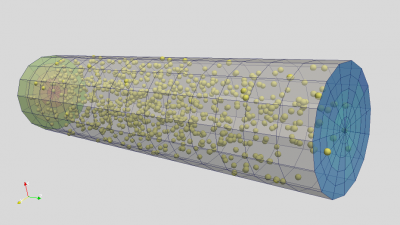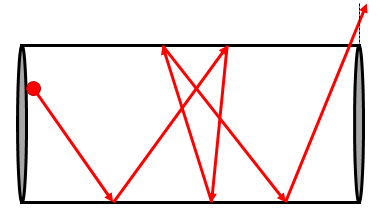# Free molecular flow in a cylindrical pipe – with multithreadingPosted on March 15th, 2016
Previous Article :: Next ArticleYou may have noticed many recent blog posts dealing with “contamination modeling”. While I started my scientific computing career with plasma simulations, these days much of my focus (and work projects) is spent on modeling contaminant transport. It’s like PIC, but without all the complicated plasma physics! There are two types of contaminants that are of interest: molecular and particulate. When it comes to molecular transport, designer of some sensitive sensor may want to know how much deposition to expect when placed in a vacuum chamber. Coming up with the answer requires computing gray body view factors between the target and all sources of contaminants. There are two ways of going about this: solving a system of linear equations, or performing Monte Carlo ray tracing. The ray tracing approach shares many similarities with PIC: we use sources to inject simulation particles, integrate their positions, perform surface interactions, and compute surface flux to objects of interest.

While this sounds simple, the devil is in the details. In this case, the detail is the surface interaction. The sensitive component will often be located outside a direct line of sight of sources, and it may take a molecule many bounces before it randomly finds its way to the sensor. Hence it is crucial that your code models these bounces correctly. Molecules reflecting from a rough surface bounce off such that flux follows cosine distribution about the surface normal. It’s quite easy to implement this diffuse reflection incorrectly! A common test is to consider conductance, or specifically transmission probability, of molecules through a cylindrical pipe. This problem was first addressed by Clausing (in 1932!). Clausing  tabulated his closed form formula for several simple geometries, including a pipe of varying L/D (length over diameter) ratios. Other researchers later came up with their own values for the “Clausing factor”, including DeMarcus and Berman. This latter data is tabulated in O’Hanlon . The two solutions are very close but diverge slightly once L/D exceeds 5, as can be seen in Figure 1.

## Simulation Code

In this article we’ll write a code for computing these transmission factors for free molecular flow in a cylindrical pipe. The pipe is defined analytically (as opposed to using a surface mesh), allowing us to consider arbitrary L/D ratios. The diameter D is set to 1, hence we simply vary the pipe length L. The pipe consists of three surfaces: inlet, wall, and outlet. The simulation proceeds as follows:

1. Particles are continuously injected at the inlet at random position and velocity following the cosine law
2. If a particle hits the wall, it is reflected from the point of impact with new velocity also sampled from the cosine law
3. If a particle crosses the inlet or the outlet, the particle is removed and the appropriate counter inlet or outlet is incremented
4. Simulation runs for a total of million time steps. Transmission factor is computed as outlet/(inlet+outlet)

The main loop is shown below:

    /*main loop, runs for num_ts time steps*/
void mainLoop()
{
for (ts=0;ts<num_ts;ts++)
{
InjectParticles();
MoveParticles();
}

finished = true;
}

Below is the code for injecting particles. It uses lambertianVector function to sample a random unit vector about normal with the two tangents given by tang1 and tang2.

    /*injects np_per_ts particles at z=0 with cosine distribution about z axis*/
void InjectParticles()
{
double source_n = {0,0,1};     //source normal direction
double tang1 = {1,0,0};        //tangent 1
double tang2 = {0,1,0};        //tangent 2

for (long p=0;p<np_per_ts;p++)
{
double pos;
double vel;
double rr;

//select random position between [-R:R]x[-R:R], accept if in tube
do {
pos=R*(-1+2*rnd());
pos=R*(-1+2*rnd());

rr= pos*pos+pos*pos;
if (rr<=R*R) break;
} while(true);

pos=0;    //z=0

//velocity
double n;
lambertianVector(n,source_n,tang1,tang2);

//multiply by drift velocity
vel= n*vdrift;
vel= n*vdrift;
vel= n*vdrift;

particles.push_back(new Particle(pos,vel));
}
}The particle mover is shown below. Particles are stored in a C++ linked list data structure. This data structure makes it easy to remove entries (killed particles) but is not as efficient to traverse as the consecutive array. We iterate through the particles using an iterator. Erasing a particle returns a new iterator and invalidates the existing one, hence the need for the while loop. The iterator is incremented for alive particles with it++. The code structure should be familiar to anyone having taken my PIC Fundamentals course. We check for wall impact by computing $$r=\sqrt{x^2+y^2}$$. If $$r>R$$, the cylinder radius, the particle hit a wall. We also check for particles leaving through inlet or the outlet by making sure that $$z\in(0,L)$$. We check for wall impact first, otherwise the particle in the sketch above may be counted as leaving the tube on the final bounce. If a particle hit the wall, the parametric position of the impact is computed from t=(R-r0)/(r-r0) where r0 is the radial distance of the particle at the start of the push. The particle is then pushed for the remaining dt_rem.

    /*moves particles, reflecting ones hitting the tube wall*/
void MoveParticles()
{
double x_old;

list<Particle *>::iterator it = particles.begin();
double sum=0;

while (it!=particles.end())
{
Particle *part = *it;
double dt_rem = dt;

//while there is delta t left in particle push
while(dt_rem>0)
{
//save old position
vec::copy(x_old,part->pos);

//new position, x = x + v*dt

//did particle hit the wall?
double rr = part->pos*part->pos+part->pos*part->pos;
if (rr>=R*R)
{
//compute intersection point
double r = sqrt(rr);
double r0 = sqrt(x_old*x_old+x_old*x_old);
double t = (R-r0)/(r-r0);

//push particle to the wall

//update remaining delta_t
dt_rem -= t*dt_rem;

//surface normal vector
double normal;
normal = 0-part->pos;
normal = 0-part->pos;
normal = 0;

vec::unit(normal,normal);

//get tangents
double tang1, tang2;
tangentsFromNormal(normal,tang1,tang2);

//cosine emission
double dir;
lambertianVector(dir, normal, tang1, tang2);
for (int i=0;i<3;i++)
part->vel[i]=dir[i]*vdrift;
} //dt_rem
else //no wall impact
{
//particle left through inlet or outlet?
if (part->pos<=0 || part->pos>=L)
{
if (part->pos<0)
inlet++;
else
outlet++;
//kill particle
delete part;
it = particles.erase(it);
break;        //break out of dt_rem loop
}
else    //particle inside the cylinder
{
dt_rem = 0;
it++;    //go to next particle
}
}
} //while dt_rem
}    //particle loop
}

Below is the “meat” of the simulation, the code that samples a random vector according to the cosine law.

    /*returns direction from cosine distribution about normal given two tangents*/
void lambertianVector(double dir, double normal, double tang1, double tang2)
{
//sample angle from cosine distribution
double sin_theta = sqrt(rnd());     //sin_theta = [0,1)
double cos_theta = sqrt(1-sin_theta*sin_theta);

double phi = 2*PI*rnd();

double vn,vt1,vt2;
vec::mult(vn,normal,cos_theta);
vec::mult(vt1,tang1,sin_theta*cos(phi));
vec::mult(vt2,tang2,sin_theta*sin(phi));

for (int i=0;i<3;i++)
dir[i] = vn[i]+vt1[i]+vt2[i];
}

The lambertianVector function requires besides the normal also two tangents. For a cylinder, there is probably a closed form for the tangents. However, the below code also works. It creates two randomly oriented tangent vectors from an arbitrary normal. It does this by first finding the spatial direction in which the normal has the largest component. It then creates a test vector in the “following” direction. As I am not using the right syntax, I am sure this is quite confusing. If the normal is mainly in the y direction, the test vector will be in the z direction. The two vectors are then crossed, giving us the first tangent, since the result of a cross-product is perpendicular to the two arguments. The second tangent is computed by crossing the first tangent with the normal.

    /*generates two tangent vectors, assumes normalized input*/
void tangentsFromNormal(double n, double tang1, double tang2)
{
//get maximum direction of the normal
int max_i=0;
double max = abs(n);
for (int i=1;i<3;i++) if (abs(n[i])>max) {max=abs(n[i]);max_i=i;}

//create a test vector that is not parallel with norm
double test = {0,0,0};
if (max_i<2) test[max_i+1]=1.0; else test=1.0;

//cross the two vectors, this will give the first tangent -perpendicular to both
vec::cross(tang1,n,test);    //assuming n is already normalized

//and the second vector//
vec::cross(tang2,n,tang1);
}

/*conductance class*/
class ConductanceXYZ
{
public:
const double L;          //cylinder length

const long np_per_ts;    //number of particles to create per time step
const int num_ts;        //number of timesteps to run

const double vdrift = 5;    //injection velocity
list<Particle*> particles;  //list of particles

const double dt=1e-2;    //simulation time step size
int ts;        //current time step

int inlet = 0;    //number of particles crossing inlet
int outlet = 0;   //number of particles crossing outlet

bool finished = false;    //set true once loop finishes

/*constructor, initializes parameters and seeds random number generator*/
ConductanceXYZ(double L, long np_per_ts, int num_ts):
gen((unsigned int)chrono::system_clock::now().time_since_epoch().count()),
L(L), np_per_ts(np_per_ts), num_ts(num_ts)    {
}

static void start(ConductanceXYZ *p)   {p->mainLoop();}

/*random number generator*/
mt19937 gen;
double rnd() {
uniform_real_distribution<double> rnd_dist(0.0,1.0);
return rnd_dist(gen);
}
//	...
}

#include <iostream>
#include <iomanip>
#include <random>
#include <list>
using namespace std;
//...

/*********   MAIN *********/
int main(int nargs, char*args[])
{

double L=1;
double part_totf = 1e7;	//double so we can use scientific notation

//get parameters from command line if specified
if (nargs>1) L=atof(args);
if (nargs>3) part_totf=atof(args);

//show active parameters

long part_tot = (long)part_totf;
int num_ts = 1000000;	//number of time steps

if (np_per_ts<1) np_per_ts=1;	//make sure we get at least 1

clock_t start = clock();    //start timer

list<ConductanceXYZ> sims;    //storage for our simulations

sims.emplace_back(L,np_per_ts, num_ts);

bool finished;
do
{
long tot_gen = 0;
long tot_out = 0;
long tot_np = 0;
int ts = num_ts+1;
finished = true;
//combine counts across threads and also check for completion
for (ConductanceXYZ &sim:sims) {
tot_gen += sim.inlet + sim.outlet;
tot_out += sim.outlet;
tot_np += sim.particles.size();
if (sim.ts<ts) ts=sim.ts;
finished &= sim.finished;    //boolean and, any false will clear it
}
double K=0;
if (tot_gen>0) K=tot_out/(double)(tot_gen);
cout<<"it="<<ts<<"\t np="<<setprecision(4)<<tot_np<<"\t K="<<K<<endl;

//sleep for 50 milliseconds
} while (!finished);

clock_t end = clock();
cout<<"Simulation took "<<(end-start)/((double)CLOCKS_PER_SEC)<<" seconds"<<endl;
return 0;
}